StatLect

Sample point

In a probabilistic experiment, a sample point is one of the possible outcomes of the experiment. The set of all sample points is called sample space.Notation

The sample space is usually denoted by the Greek letter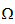(Omega) and a sample point is indicated by its lowercase version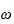(omega):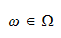Example

Suppose the random experiment is the flip of a coin. It can give rise to two possible outcomes: tail (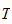) and head (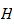).andare the two sample points and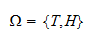is the sample space.

More details

The notions of sample space and sample point are discussed in detail in the lecture entitled Probability.